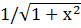### Sample Problem

Write an algebraic expression that is equivalent to sec(arcsin).

#### SolutionLet arcsin ().= u

Therefore, sin u=and by the Pythagorean Theorem, we know that the last side of the triangle=x.

Sec u= 1/cos u=1/(adj/hyp)=hyp/adj=√1+x2/x